• Anonymous (not verified) 10 years 28 weeks ago

• 10 years 30 weeks ago

Here is a point which I feel needs to be emphasized more in Example 3.

If we try to make the argument work without leaving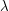to be optimized later, but by, say, initially setting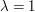, the argument will fail: we will get a useless bound. The reason: we need to pick a function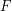which will grow rapidly while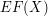will grow slowly. In this case,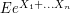grows pretty fast with.

What kind of functionswork for this? Well,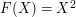works: out of the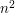terms in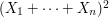, almost all have expectation. Similarly,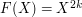works fine as well: in the sum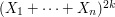, we will be able to easily cancel many terms because they have expectation.

The central insight behind the proof in Example 3 is that picking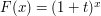for really, really smallworks. The reason: the average of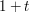and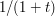is really close to. That is: near one, inversion is about the same as subtraction. This insight can be pushed a little further to argue that if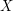has mean, the quantity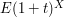should be pretty close to. Thus one might hope that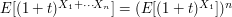grows slowly - after all, it is "approximately"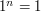.

• Anonymous (not verified) 10 years 37 weeks ago

Maybe it'd be nice to mention that if your space is being decomposed into U and V and these have non-empty intersection, then the end terms (H_0 and such) can be taken as reduced homology instead of just normal homology, simplifying some calculations.

• Anonymous (not verified) 10 years 37 weeks ago

If I recall correctly, the Mayer-Vietoris sequence tells you something about a space decomposed as two sets _whose interiors cover the space_. So I think U and V must intersect in two intervals (which are homotopically trivial, so the rest of the proof runs the same).

• Anonymous (not verified) 10 years 38 weeks ago

what is uv?

• Nicolai H (not verified) 10 years 42 weeks ago Dimension arguments in combinatorics

I would suggest using the less eponymous term "Shatter function lemma" (that Matousek uses in his Lectures on Discrete Geometry) in addition to "Sauer-Shelah lemma". I personally have known this lemma for a long time, but always under the more descriptive name "Shatter function lemma".

• Marius Overholt (not verified) 10 years 45 weeks ago Smoothing sums

It should be Acta Arithmetica, of course!

• Marius Overholt (not verified) 10 years 45 weeks ago Smoothing sums

It was actually Waclaw Sierpinski who obtained the exponent 1/3 in the Gauss circle problem, as part of his doctoral dissertation, and who published the result in 1906 (in Polish). His adviser Georgy Voronoi had obtained the same exponent in the Dirichlet divisor problem in 1903. There is an article by Andrzej Schinzel about Sierpinski's papers in number theory in volume XXI of Acta Mathematica (1972). Also there is supposed to exist (but which I have never seen) a description of Sierpinski's proof in German, possibly in some Jahrbuch der Deutsche Mathematikerverein from those years. Schinzel states that the proof is by Voronoi's geometric method. That would mean that the circle is approximated by a polygon, and Euler-Maclaurin summation applied on each piece.

• maexe (not verified) 10 years 50 weeks ago

In Proof of Theorem 2:
... use Theorem 1 to identify \mathrm{Hom}_{k(G)}(U,U \otimes_k V) with V (not U),
we see that we may regard S as a subspace of V (not U).
Beyond this, a very nice article.

• Dia Sharma (not verified) 10 years 51 weeks ago

Very good but it would be nice if variable seperation method would be included.

• 11 years 3 days ago Complete the square

I don't find the article unintelligible at all; perhaps you could clarify what you find problematic.

Also, editing a page to correct typos (like the missing coefficient you spotted) is very simple: just click on edit at the top of the article. I've corrected it here.

• 11 years 6 days ago Complete the square

How can it be possible to transform the method of completing the square into such a "charabia".

By the way, a(x+x_0)^2 = ax^2 + 2ax x_0 + x_0^2 is false.

• 11 years 1 week ago

This should probably be restructured to match the form of the articles under "What kind of problem am I trying to solve?". I might even do so myself if I have the time and energy...

• 11 years 1 week ago

This describes one of the most fundamental and general mathematical techniques there is, one so basic that probably most math majors find it second nature already. As such, the description of it as a general technique may be most useful for lower-level pedagogy; failing to grasp this general technique can make math very hard for primary and secondary school students. However, I would love to have examples of its use at all levels and fields, since it is so general-purpose.

• 11 years 1 week ago

Blech, yes, I got stuff reversed. Fixing.

• 11 years 1 week ago

It's a Wiki. Usual advice is to rewrite the style yourself. :-)

• Anonymous (not verified) 11 years 2 weeks ago

My personal favorite way of understanding cubics is the following. In analogy to quadratic equations, try a solution of the form u + v, figuring once we get a single root we can get the other two in short order. Then get rid of the bx^2 term like you did, obtaining x^3 + cx + d = 0. The idea now is to cancel the cx term too. Namely, x^3 = u^3 + 3uv(u + v) + v^3, and cx = c(u + v), so to cancel the cx
term and the 3uv(u + v) term at the same time one stipulates that 3uv = -c.

As a result, one has two equations now: u^3 + v^3 + d = 0, and 3uv = -c. Plugging
v = -c/3u into the first equation gives a quadratic equation in u^3, which has
six solutions u. To each of these three is a single v = -c/3u that works and then one obtains six solutions u + v. But of course u and v are symmetric here so we really just have 3 solutions.

• 11 years 3 weeks ago The tensor power trick

For consistency I've now decided to use \chi throughout that section.

• Anonymous (not verified) 11 years 4 weeks ago The tensor power trick

Before (1), the equation for the Fourier transform has \chi when it should have \xi.

• 11 years 5 weeks ago

I don't think one really needs to apply the axiom of choice in this context: Since the multifunction is taking values in positive integers, we could just define the corresponding function by taking its value to be the least member of the set of values of the multifunciton. (This process will then, I guess, secretly take us back to the original function construction, where we write each rational in lowest terms — but we don't need to pay attention to this if we don't want to.)

• Anonymous (not verified) 11 years 5 weeks ago

• Michael Burge (not verified) 11 years 5 weeks ago

I like how you snuck in the Axiom of Choice here, and hid it under the rug since its use is "clear".

• Anonymous (not verified) 11 years 7 weeks ago

Should "difficult" be changed to "easy"?

• Didier Piau (not verified) 11 years 9 weeks ago How to use martingales

There is a typo in the second paragraph of section Example 1, continued: one should cancel the symbol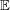in the two occurrences of

the expected value of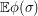given that

And, reading the last paragraph of this section, I wondered why you picked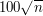as an example of extremely unlikely deviation. First,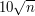would already yield a rather respectable (i.e., small) bound, namely, something like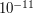. Second, there is nothing specific abouthere, although the formulation seems to indicate otherwise: you might want to add a for example somewhere.